Question

# (1 point) A first order linear equation in the form y' + p(x)y = f(x) can...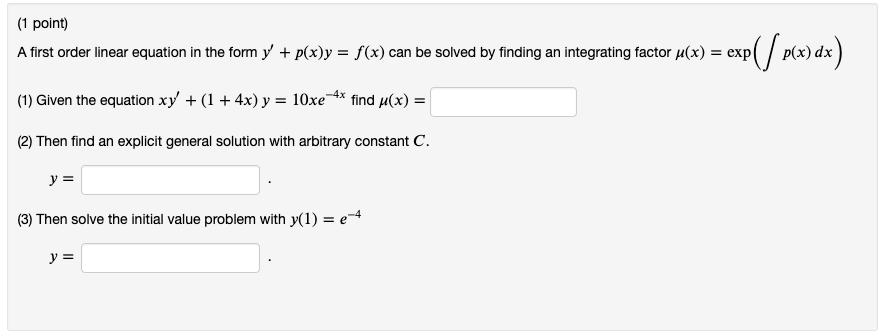(1 point) A first order linear equation in the form y' + p(x)y = f(x) can be solved by finding an integrating factor u(x) = expl (1) Given the equation xy' + (1 +4x) y = 10xe 4* find y(x) = (2) Then find an explicit general solution with arbitrary constant C. y = (3) Then solve the initial value problem with y(1) = e-4 y =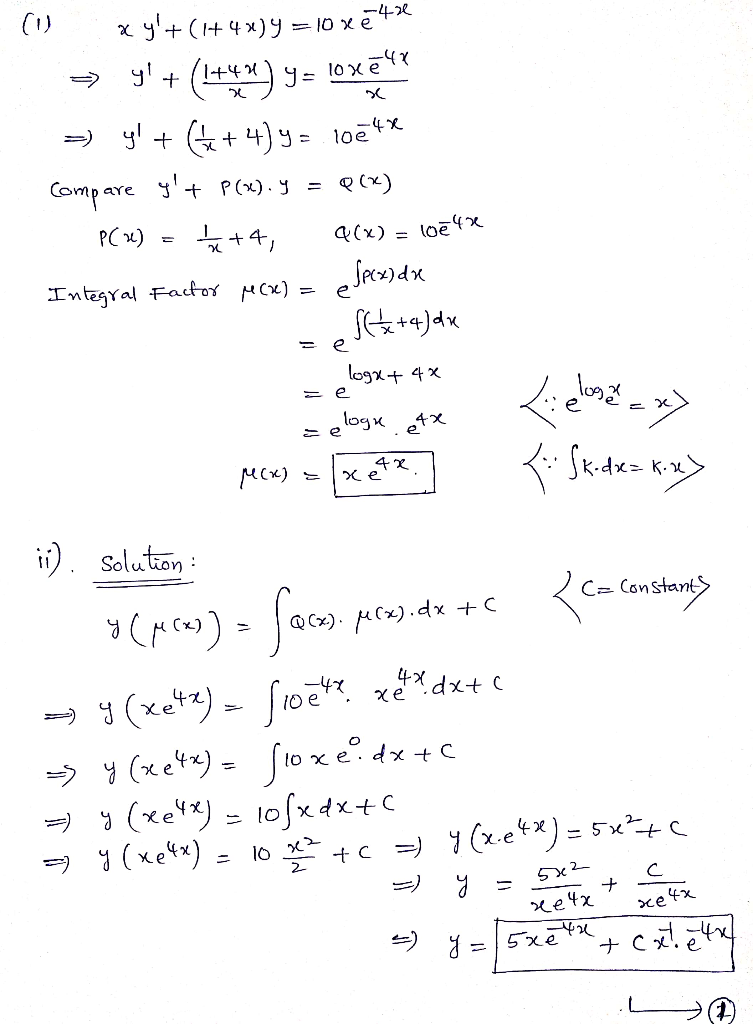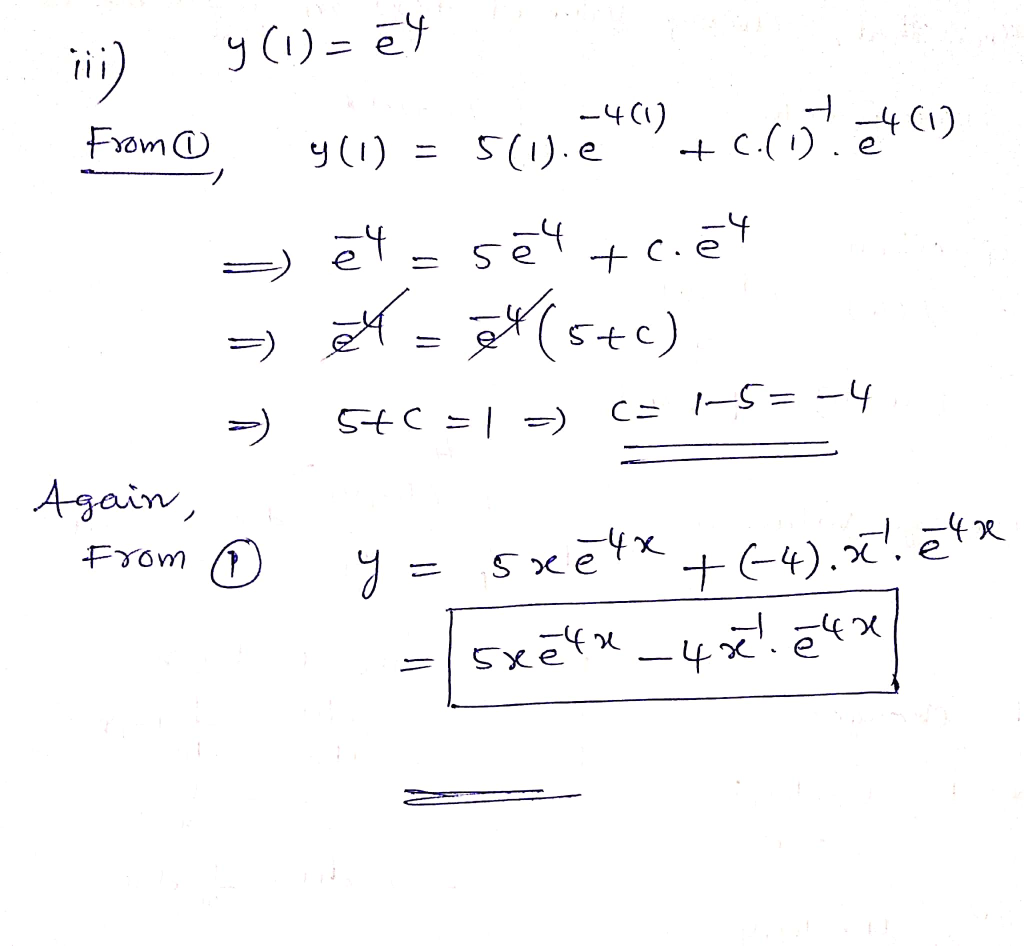#### Earn Coins

Coins can be redeemed for fabulous gifts.

Similar Homework Help Questions
• ### (1 point) A first order linear equation in the form y +p(x)y -f(x) can be solved...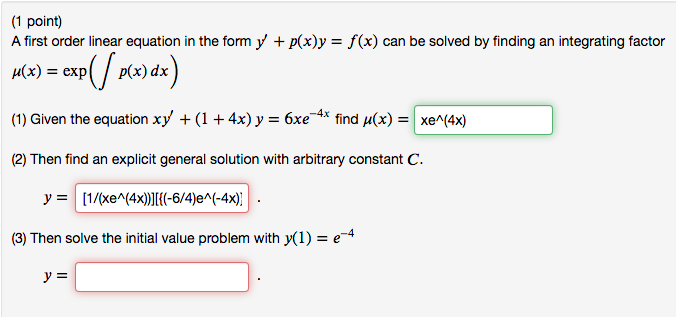(1 point) A first order linear equation in the form y +p(x)y -f(x) can be solved by finding an integrating factor H(x)exp /p(x) dx (1) Given the equation xy + (1 + 4x) y-6xe_4x find (x)-| xeN4x) (2) Then find an explicit general solution with arbitrary constant C (3) Then solve the initial value problem with y(1)e

• ### A first order linear equation in the form y p(x)y = f(x) can be solved by...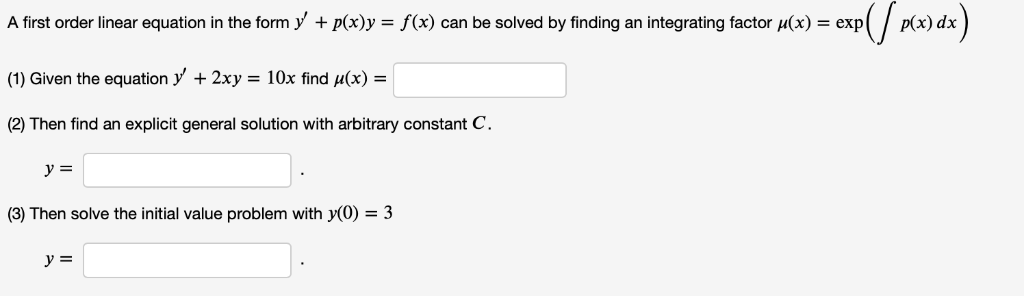A first order linear equation in the form y p(x)y = f(x) can be solved by finding an integrating factor u(x) = exp c) dx (1) Given the equation y 2xy = 10x find H(x) = (2) Then find an explicit general solution with arbitrary constant C у %3 (3) Then solve the initial value problem with y(0) = 3 A first order linear equation in the form y p(x)y = f(x) can be solved by finding an integrating factor...

• ### A first order linear equation in the form y' + pay = f() can be solved...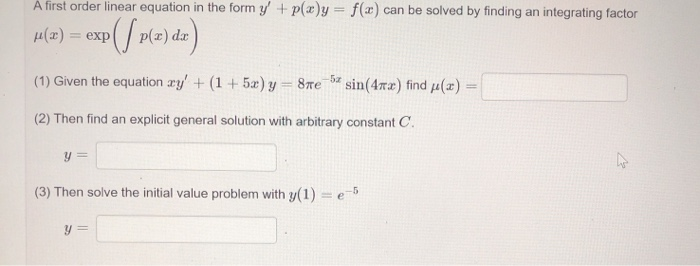A first order linear equation in the form y' + pay = f() can be solved by finding an integrating factor H(x) = exp() P(a) dx) (1) Given the equation xy' + (1 + 5x) y = 8e 5 sin(4x) find () = (2) Then find an explicit general solution with arbitrary constant C. y = (3) Then solve the initial value problem with y(1) = e-5

• ### (1 point) A first order linear equation in the form y' + p(x)y = f(x) can...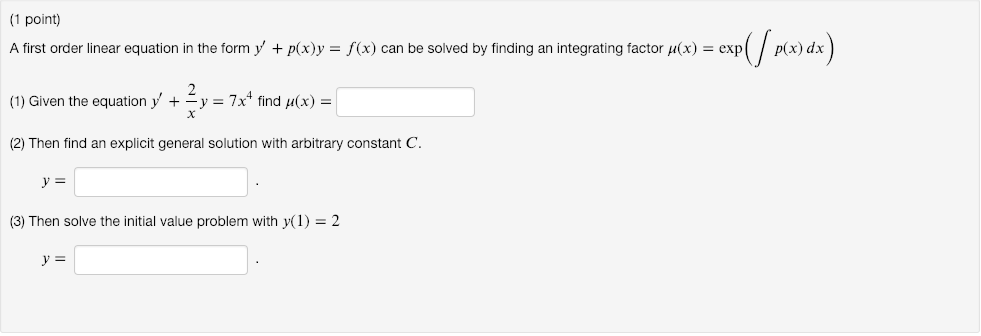(1 point) A first order linear equation in the form y' + p(x)y = f(x) can be solved by finding an integrating factor μ(x) = exp ( (1) Given the equation y, +-= 7x4 find μ(x) (2) Then find an explicit general solution with arbitrary constant C p(x) dx (3) Then solve the initial value problem with y(1) = 2

• ### (1 point) A first order linear equation in the form y' + p(x)y = f(x) can...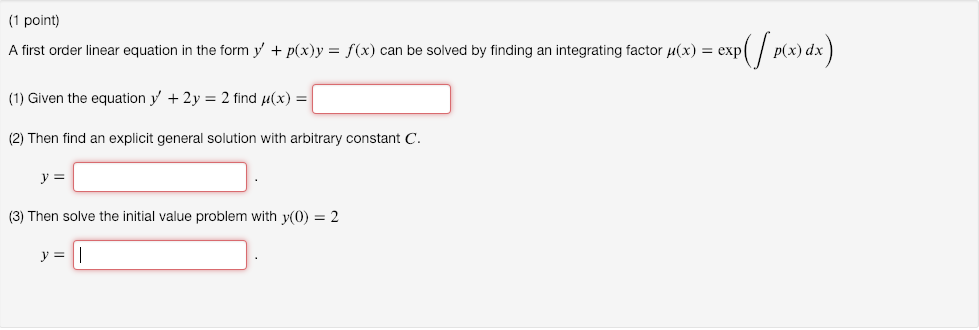(1 point) A first order linear equation in the form y' + p(x)y = f(x) can be solved by finding an integrating factor μ(x) = exp (1) Given the equation y' + 2y = 2 find μ(x) (2) Then find an explicit general solution with arbitrary constant C p(x) dx (3) Then solve the initial value problem with y(0) 2

• ### (1 point) A first order linear equation in the form y' + p(x) = f(x) can...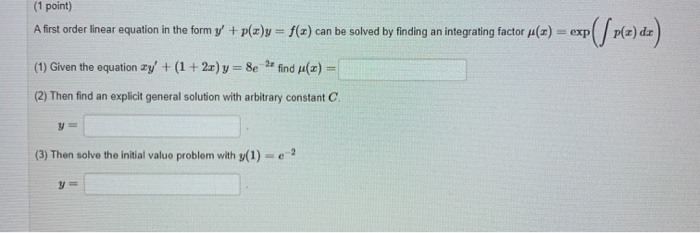(1 point) A first order linear equation in the form y' + p(x) = f(x) can be solved by finding an integrating factor (1) exp(/ pla) de) (1) Given the equation ay' + (1 + 2x) y = 8e 22 find (x) (2) Then find an explicit general solution with arbitrary constant C (3) Then solve the initial value problem with y(1) - ?

• ### (1 point) A first order linear equation in the form y p(x)yf(x) can be solved by...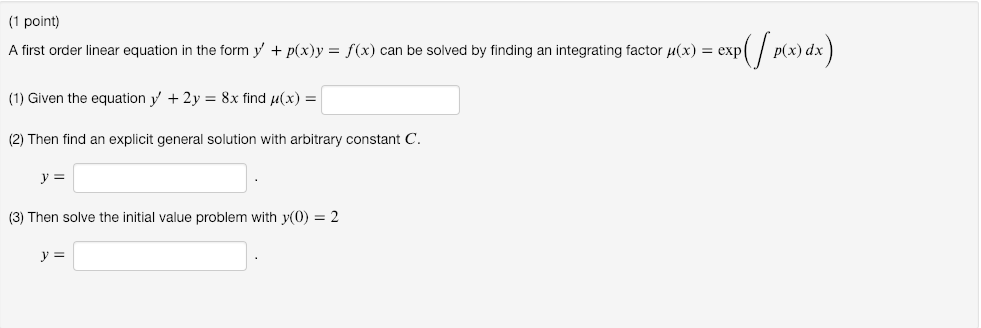(1 point) A first order linear equation in the form y p(x)yf(x) can be solved by finding an integrating factor x)expp(x) dx (1) Given the equation y' +2y-8x find u(x) - (2) Then find an explicit general solution with arbitrary constant C. (3) Then solve the initial value problem with y(0) 2 y-

• ### A first order linear equation in the form y′+p(x)y=f(x) y p x y f x can be solved by finding an integrating factor μ(x)=...

A first order linear equation in the form y′+p(x)y=f(x) y p x y f x can be solved by finding an integrating factor μ(x)=exp(∫p(x)dx) μ x exp p x d x (1) Given the equation y′+6y=4 y 6 y 4 find μ(x)= μ x (2) Then find an explicit general solution with arbitrary constant C C . y= y . (3) Then solve the initial value problem with y(0)=3 y 0 3 y= y .

• ### 1 point) An equation in the form y + p(x)y -(x)y with n 0, 1 is...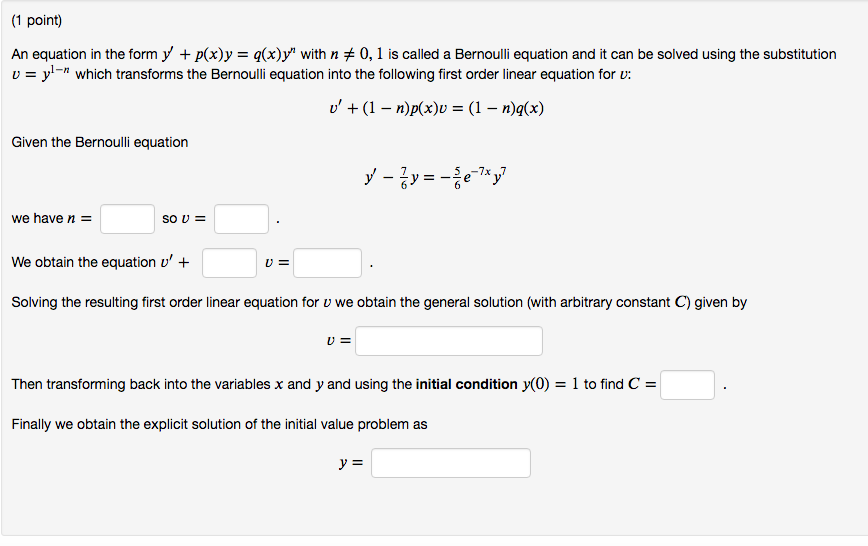1 point) An equation in the form y + p(x)y -(x)y with n 0, 1 is called a Bernoulli equation and it can be solved using the substitution wich transforms the Bernoulli equation into the following first order linear equation for v: Given the Bernoulli equation we have n- We obtain the equation u' Solving the resulting first order linear equation for v we obtain the general solution (with arbitrary constant C) given by Then transforming back into the variables...

• ### (1 point) The equation 3ry2r 2y2 (*) can be written in the form y f(y/x), ie.,...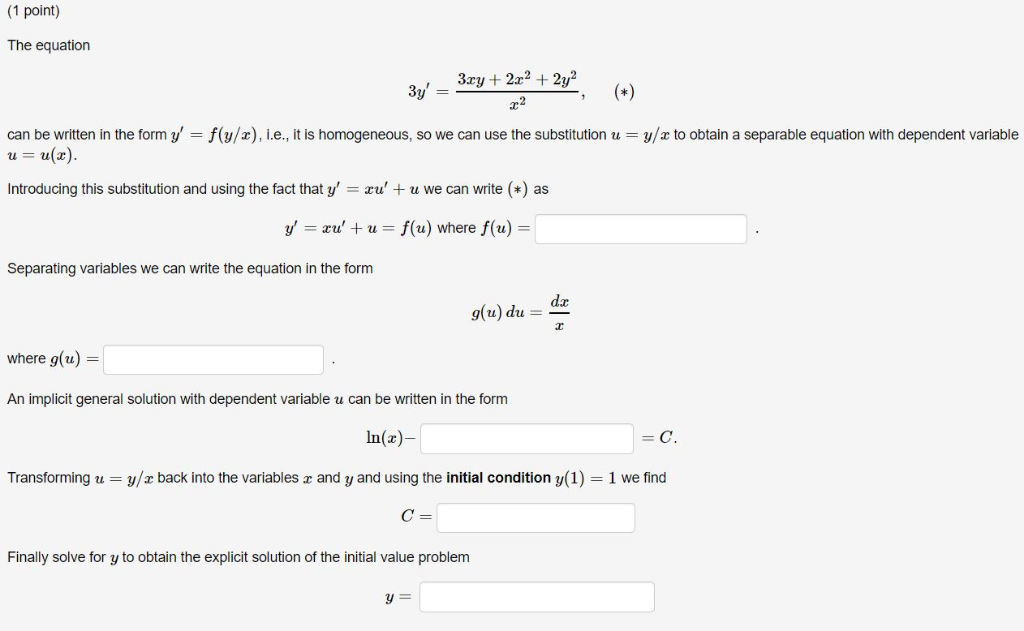(1 point) The equation 3ry2r 2y2 (*) can be written in the form y f(y/x), ie., it is homogeneous, so we can use the substitution u = y/x to obtain a separable equation with dependent variable uu(x. Introducing this substitution and using the fact that y' ru' u we can write () as y xu'w = f(u) where f(u) Separating variables we can write the equation in the form da np (n)6 where g(u) = An implicit general solution with...